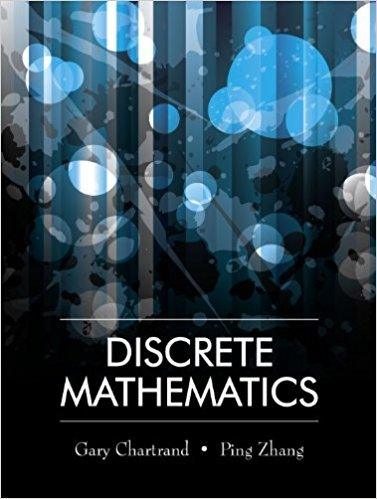×
×

# Solutions for Chapter 15.3: Finite-State Machines## Full solutions for Discrete Mathematics | 1st Edition

ISBN: 9781577667308Solutions for Chapter 15.3: Finite-State Machines

Solutions for Chapter 15.3
4 5 0 382 Reviews
24
3
##### ISBN: 9781577667308

This textbook survival guide was created for the textbook: Discrete Mathematics, edition: 1. Since 20 problems in chapter 15.3: Finite-State Machines have been answered, more than 12315 students have viewed full step-by-step solutions from this chapter. This expansive textbook survival guide covers the following chapters and their solutions. Chapter 15.3: Finite-State Machines includes 20 full step-by-step solutions. Discrete Mathematics was written by and is associated to the ISBN: 9781577667308.

Key Math Terms and definitions covered in this textbook
• Change of basis matrix M.

The old basis vectors v j are combinations L mij Wi of the new basis vectors. The coordinates of CI VI + ... + cnvn = dl wI + ... + dn Wn are related by d = M c. (For n = 2 set VI = mll WI +m21 W2, V2 = m12WI +m22w2.)

• Complex conjugate

z = a - ib for any complex number z = a + ib. Then zz = Iz12.

A sequence of steps (end of Chapter 9) to solve positive definite Ax = b by minimizing !x T Ax - x Tb over growing Krylov subspaces.

• Determinant IAI = det(A).

Defined by det I = 1, sign reversal for row exchange, and linearity in each row. Then IAI = 0 when A is singular. Also IABI = IAIIBI and

• Diagonalizable matrix A.

Must have n independent eigenvectors (in the columns of S; automatic with n different eigenvalues). Then S-I AS = A = eigenvalue matrix.

• Dimension of vector space

dim(V) = number of vectors in any basis for V.

• Elimination.

A sequence of row operations that reduces A to an upper triangular U or to the reduced form R = rref(A). Then A = LU with multipliers eO in L, or P A = L U with row exchanges in P, or E A = R with an invertible E.

• Left nullspace N (AT).

Nullspace of AT = "left nullspace" of A because y T A = OT.

• Length II x II.

Square root of x T x (Pythagoras in n dimensions).

• Minimal polynomial of A.

The lowest degree polynomial with meA) = zero matrix. This is peA) = det(A - AI) if no eigenvalues are repeated; always meA) divides peA).

• Nullspace matrix N.

The columns of N are the n - r special solutions to As = O.

• Permutation matrix P.

There are n! orders of 1, ... , n. The n! P 's have the rows of I in those orders. P A puts the rows of A in the same order. P is even or odd (det P = 1 or -1) based on the number of row exchanges to reach I.

• Pivot columns of A.

Columns that contain pivots after row reduction. These are not combinations of earlier columns. The pivot columns are a basis for the column space.

• Rank one matrix A = uvT f=. O.

Column and row spaces = lines cu and cv.

• Schwarz inequality

Iv·wl < IIvll IIwll.Then IvTAwl2 < (vT Av)(wT Aw) for pos def A.

• Special solutions to As = O.

One free variable is Si = 1, other free variables = o.

• Toeplitz matrix.

Constant down each diagonal = time-invariant (shift-invariant) filter.

• Trace of A

= sum of diagonal entries = sum of eigenvalues of A. Tr AB = Tr BA.

• Triangle inequality II u + v II < II u II + II v II.

For matrix norms II A + B II < II A II + II B II·

• Tridiagonal matrix T: tij = 0 if Ii - j I > 1.

T- 1 has rank 1 above and below diagonal.

×